• 傅里叶反变换的原理详述，自编函数可以调用，也可以读取程序，理解算法原理MATLAB
• 离散傅里叶变换 离散傅里叶反变换
离散傅里叶变换
离散傅里叶反变换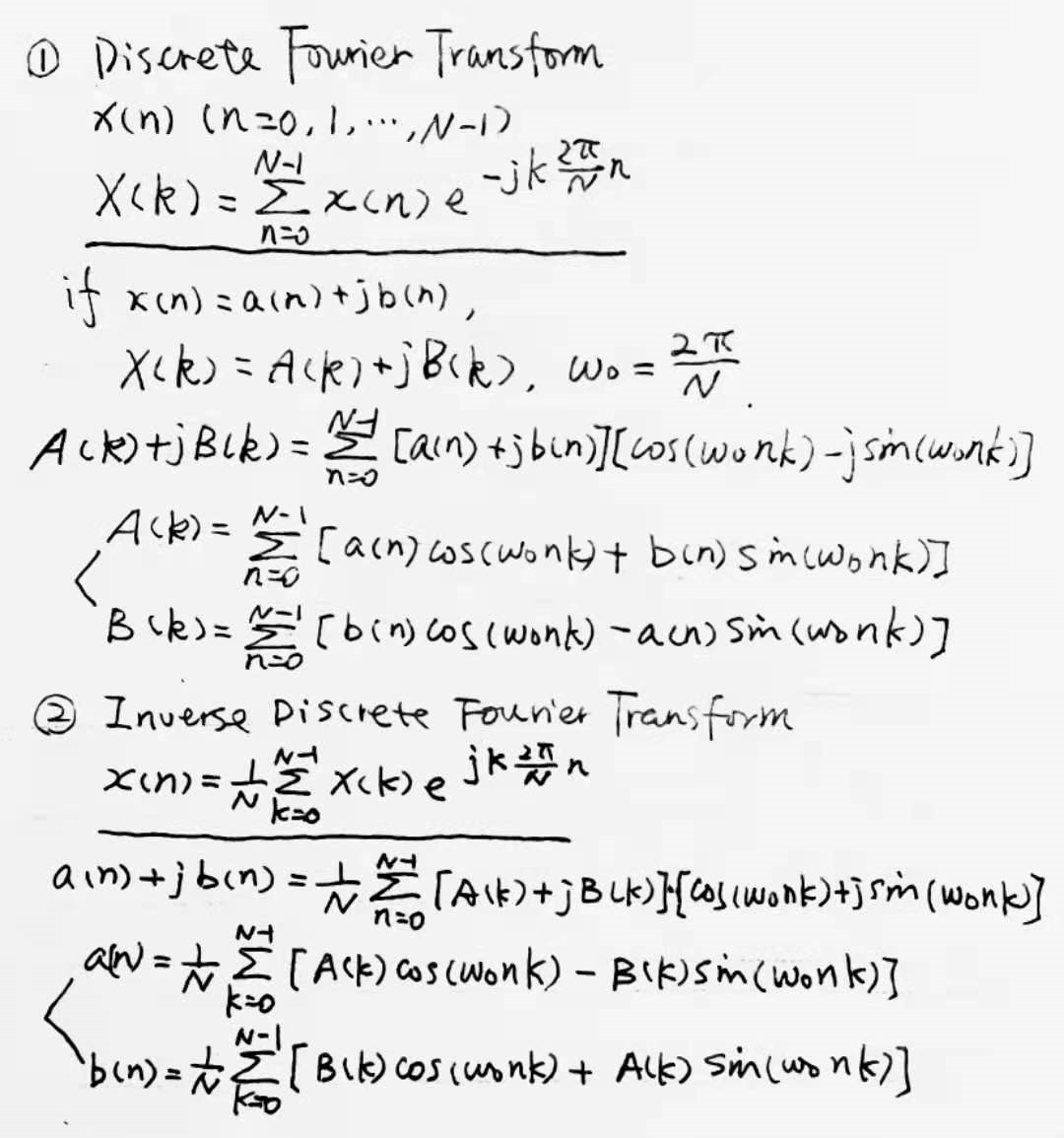傅里叶变换理论值过程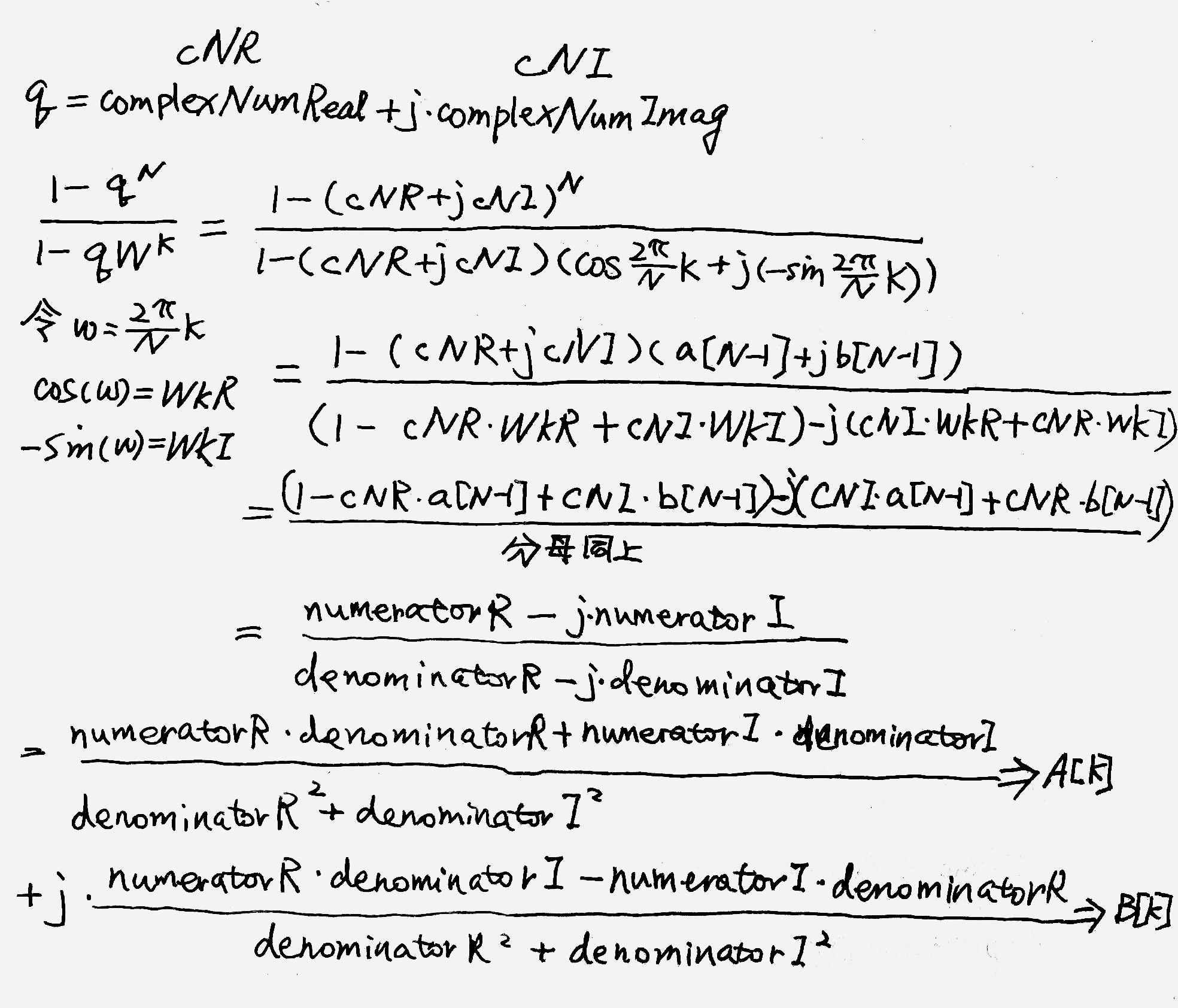dft.c
#include "math.h"
double dft(a,b,A,B,N)
int N;
double a[],b[],A[],B[];
{
double PI=acos(-1.0);
int n,k;
double c,d,w0,w,s;
w0=2*PI/N;
for(k=0;k<N;k++){
w=k*w0;
A[k]=B[k]=0.0;
for(n=0;n<N;n++){
d=n*w;
c=cos(d);
s=sin(d);
A[k]+=c*a[n]+s*b[n];
B[k]+=c*b[n]-s*a[n];
}
}
return PI;
}

idft.c
#include "math.h"
void idft(A,B,a,b,N)
int N;
double A[],B[],a[],b[];
{
double PI=acos(-1.0);
int n,k;
double c,d,w0,w,s;
w0=2*PI/N;
for(n=0;n<N;n++){
w=n*w0;
a[n]=b[n]=0.0;
for(k=0;k<N;k++){
d=k*w;
c=cos(d);
s=sin(d);
a[n]+=(c*A[k]-s*B[k])/N;
b[n]+=(c*B[k]+s*A[k])/N;
}
}
return PI;
}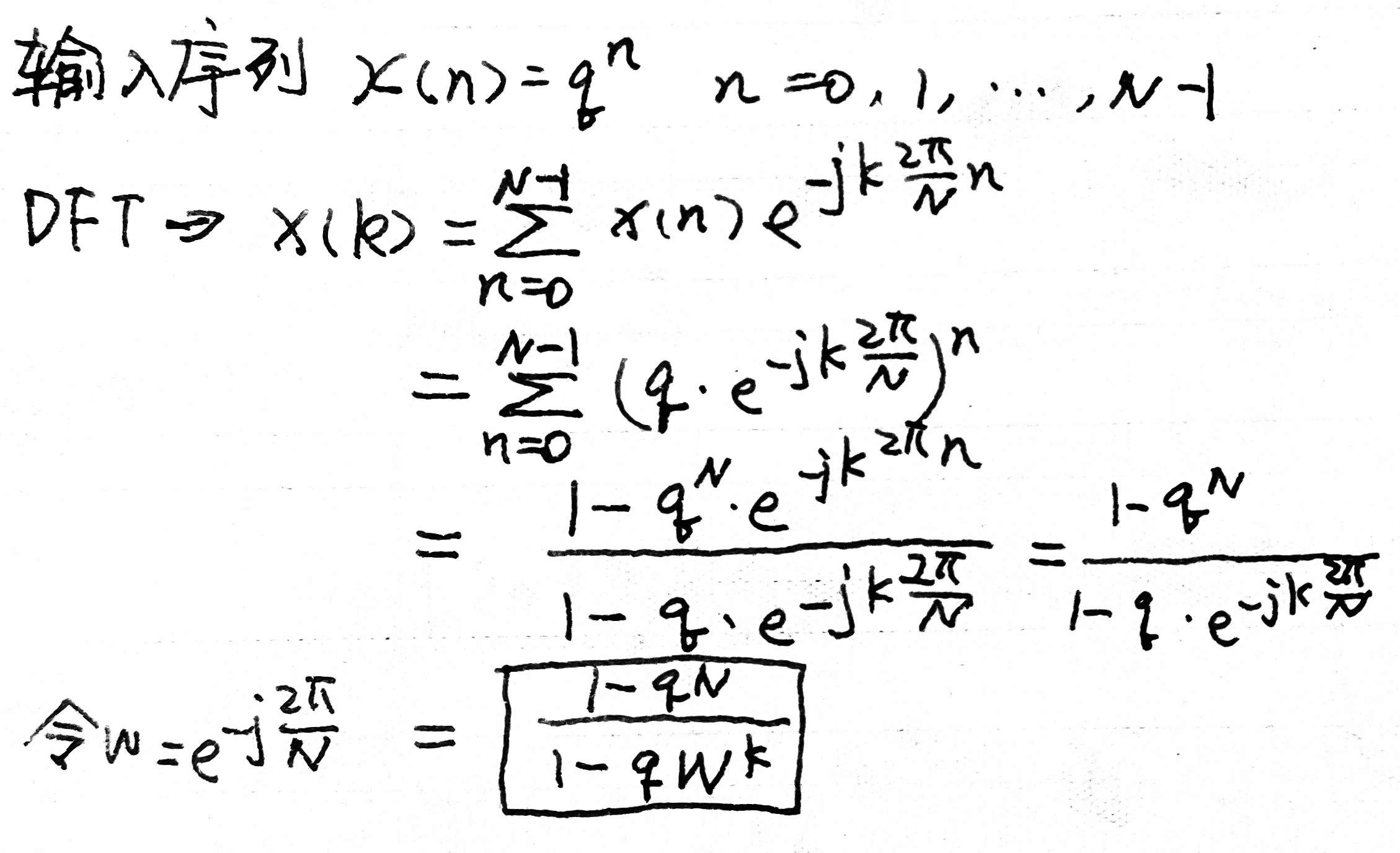其中q=complexNumReal+j*complexNumImaginary
输出x[n]，傅里叶变换实际值，理论值，并且将理论值反变换
main.c
#include "stdio.h"
#include "math.h"
#include "dft.c"
#include "idft.c"
main(){
int i,j,N;
printf("N=");
scanf("%d",&N);
double cNR,cNI;//complexNumReal,complexNumImaginary
double a[N],b[N],A[N],B[N];
printf("complexNumReal=");
scanf("%lf",&cNR);
printf("complexNumImaginary=");
scanf("%lf",&cNI);
a=1.0;
b=0.0;
for(i=1;i<N;i++){
a[i]=cNR*a[i-1]-cNI*b[i-1];
b[i]=cNI*a[i-1]+cNR*b[i-1];
}
printf("----Original Sequence----\n");
for(i=0;i<N/2;i++){
for(j=0;j<2;j++)
printf("|%d:\t%10.6f + j%10.6f|\t",2*i+j+1,a[2*i+j],b[2*i+j]);
printf("\n");
}

double PI=dft(a,b,A,B,N);
printf("----Discrete Fourier Transform----\n");
for(i=0;i<N/2;i++){
for(j=0;j<2;j++)
printf("|%d:\t%10.6f + j%10.6f |\t",2*i+j+1,A[2*i+j],B[2*i+j]);
printf("\n");
}

double nR,nI;//numeratorR,numeratorI
double dR,dI;//denominatorR,denominatorI
double w,WkR,WkI;
printf("----Theoretical Discrete Fourier Transform----\n");
for(int k=0;k<N;k++){
w=(2*PI/N)*k;   //W^k=e^-j*k*(2*PI/N)=cos(w)+j*(-1)*sin(w)
WkR=cos(w);
WkI=(-1)*sin(w);
nR=1-cNR*a[N-1]+cNI*b[N-1];
nI=cNI*a[N-1]+cNR*b[N-1];
dR=1-cNR*WkR+cNI*WkI;
dI=cNI*WkR+cNR*WkI;
A[k]=(nR*dR+nI*dI)/(pow(dR,2)+pow(dI,2));
B[k]=(nR*dI-nI*dR)/(pow(dR,2)+pow(dI,2));
}
for(i=0;i<N/2;i++){
for(j=0;j<2;j++)
printf("|%d:\t%10.6f + j%10.6f |\t",2*i+j+1,A[2*i+j],B[2*i+j]);
printf("\n");
}

idft(A,B,a,b,N);
printf("****Inverse Discrete Fourier Transform****\n");
for(i=0;i<N/2;i++){
for(j=0;j<2;j++)
printf("|%d:\t%10.6f + j%10.6f |\t",2*i+j+1,a[2*i+j],b[2*i+j]);
printf("\n");
}
}



展开全文c语言 信号处理 idft
• matlab 编写快速傅里叶反变换函数（ifft）（横向读取数据进行变换） %快速傅里叶逆变换，不够2的整数幂的个数，末尾自动补齐0
原理参考：[https://wk.baidu.com/view/da8d58d776a20029bd642d14?pcf=2]
（欢迎指正）
matlab 编写快速傅里叶反变换函数（ifft）（横向读取数据进行变换）
matlab代码：
%快速傅里叶逆变换，不够2的整数幂的个数，末尾自动补齐0
function ret_val = myifft(Vector)
%因为输入的数据可能不是2的整数次幂，补零使得计算更加方便
[m,n]=size(Vector);%输入信号矩阵大小
num=ceil(log2(n));%向上取整
N=2^num;
vector=zeros(m,N);%申请足够大小矩阵
vector(:,1:n)=Vector(:,:);%将变换后的信号输入，不足补零

for lines=1:m%循环行数
for L=num-1:-1:0%L表示运算等级或者层数
dis=2^L;%dis表示奇偶组之间的距离
for id=1:2^(num-L-1) %循环当前层数组数
%进行同址运算
for idx=1:dis%循环组内个数
x1=(id-1)*2*dis+idx;%求得奇数数组的索引值
x2=(id-1)*2*dis+dis+idx;%对应偶数数组的索引值
temp1=(vector(lines,x1)+vector(lines,x2))/2;%中间变量保存相应奇偶数组数据
temp2=(vector(lines,x1)-vector(lines,x2))/2*W(L,(x1-1));
vector(lines,x1)=temp1;%存入之前地址
vector(lines,x2)=temp2;
end
end
end
end
%变址运算，逆推回原来位置
for line=1:m%循环行数
j1 = 0;
for i = 1 : N%循环个数
if i < j1 + 1
tmp = vector(line,j1 + 1);
vector(line,j1 + 1) = vector(line,i);
vector(line,i) =tmp;
end
k = N / 2;
while k <= j1
j1 = j1 - k;
k = k / 2;
end
j1 = j1 + k;
end
end
ret_val =vector;
function val=W(L,x)%旋转因子当层数为L,索引值为x
val=exp(1j*2*pi*x/2^(L+1));
end
end

输入傅里叶变换后的结果进行反变换*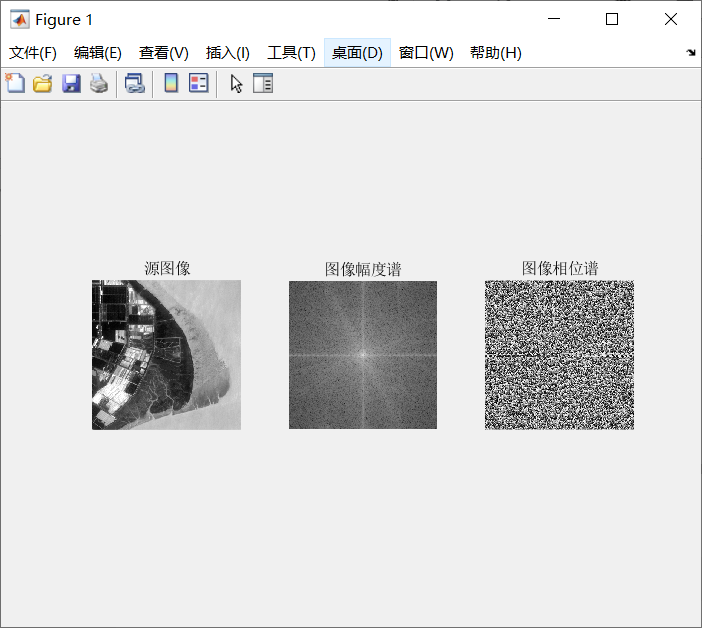反变换结果截图
右边图像右下黑框是fft变换过程中补零部分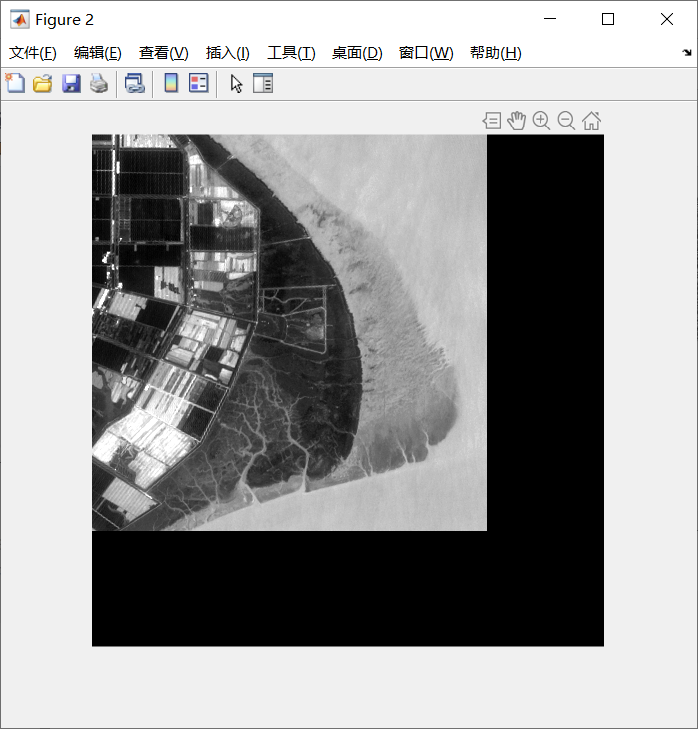当然也可以去除黑边框：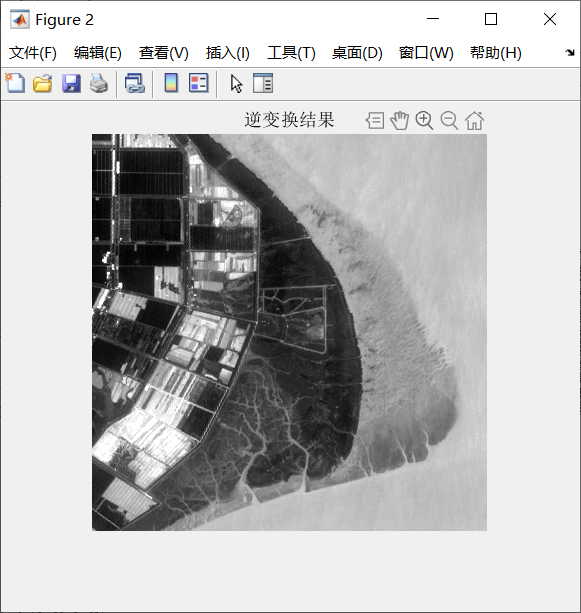说明：函数是对输入数据进行一维横向fft,对于遥感图像要进行二维变换，才能获得如图效果。
具体调用函数代码：
%快速傅里叶变换函数及逆变换函数测试程序
tic
filename='Landsat8_OLI_b1.dat';
img=cell2mat(Re(5));%获取图像矩阵参数
t1=myfft(img);%一维横向变换
f=myfft(t1.');%纵向变换
[m,n]=size(f);
[M,N]=size(img);
f=myfftshift(f);      %使图像对称,中心化
r=real(f);          %图像频域实部
i=imag(f);          %图像频域虚部
Margin=log(sqrt(r.^2+i.^2));      %图像幅度谱，加log便于显示
phase=real(angle(f)*180/pi);     %图像相位谱
figure(1)
subplot(1,3,1),imshow(linear1(img)),title('源图像');%拉伸显示
subplot(1,3,2),imshow(Margin,[]),title('图像幅度谱');
subplot(1,3,3),imshow(phase,[]),title('图像相位谱');
f=myfftshift(f);
g=myifft(f);
k=myifft(g.');
k=k(1:M,1:N);
figure(2)
imshow(linear1(k)),%拉伸显示原图像
title('逆变换结果')
toc



展开全文matlab
• 由输出信号频域，进行傅里叶反变换得到时域，应与输入信号相同，但结果并不是如此。感觉错误出现在 phi=N*T.^2/(2*pi*m); h=exp(0.5i*phi*w.^2); y2=y1.*h; x2 =ifftshift(ifft(y2)); 这几行里，求大神指导。 ...开发语言
• 根据时域圆周移位特性 可得 即X1(k)={7,2+3j,-1-2j,-j} 5.2. 6 X(k)={7,2+3j,-1-2j,-j} 5.2.3 圆周卷积定理 假设x1(n)和x2(n)经过补零到两个长度一致的有限长序列长度为N且有 则 5.2. 8 式中[x1(n) x2(n)]表示序列x1...
• 原理自己查书，代码如下： /****************************************************...功能： 一维快速傅里叶反变换 参数： complex *TD： 指向时域数组的指针 complex *FD： 指向频域数组的指针 int r： 2的幂数，即
原理自己查书，代码如下：

/****************************************************************
功能：       一维快速傅里叶反变换
参数：       complex<double> *TD： 指向时域数组的指针
complex<double> *FD：  指向频域数组的指针
int r：    2的幂数，即迭代次数
返回值：   无
***************************************************************/
void  Ctry::IFFT(complex<double> *FD, complex<double> *TD, int r)
{
LONG count;     //傅里叶变换点数
int       i;            //循环变量
complex<double> *x;
count = 1 << r;      //计算傅里叶变换点数
x = new complex<double>[count];      //分配运算所需要的存储器
//将频域点写入x
memcpy(x, FD, sizeof(complex<double>)* count);

//求共轭
for (i = 0; i < count; i++)
{
x[i] = complex<double>(x[i].real, -x[i].imag());
}
FFT(x, TD, r);     //调用快速傅里叶变换

//求时域点共轭
for (i = 0; i < count; i++)
{
TD[i] = complex<double>(TD[i].real/count, -TD[i].imag()/count);
}

delete x;   //释放内存
}

FFT函数见上一篇博客


展开全文• 在MATLAB中想要画一个sin函数是很容易的。比如:首先定义t = 0:0.01:10,然后画出y = sin(2*pi*t)就可以了，最多再加相角啊之类的参数。但如果在C语言中应该如何自己制造一个sin函数呢？应该借鉴数字信号处理里的抽样...
在MATLAB中想要画一个sin函数是很容易的。比如:首先定义t = 0:0.01:10,然后画出y = sin(2*pi*t)就可以了，最多再加相角啊之类的参数。但如果在C语言中应该如何自己制造一个sin函数呢？应该借鉴数字信号处理里的抽样思想：想生成函数x(t) = a*sin(2*pi*f0*t+ψ)，就应该考虑到抽样定理：以等时间间隔进行抽样，即x[n] = x[n*Ts] 其中Ts = 1/fs(fs是抽样频率)。这时候想生成的函数x(t)就变成了x(t) = x(n*Ts) = x(n) = a*sin(2*pi*f0*n/fs+ψ) = a*sin(w*n+ψ)，其中w是数字角频率，这就将模拟时间信号转化成了数字信号。举个例子，想生成函数y = sin(20*pi*t),用matlab直接生产的图形如下所示(取t=0:0.001：1)matlab程序为t = 0:0.001:1;y = sin(20*pi*t);plot(t,y);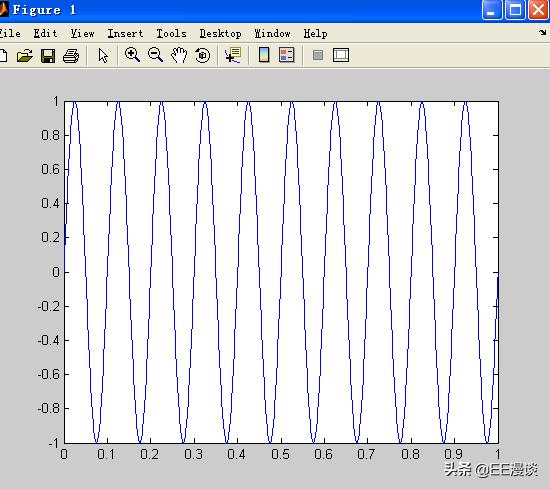如果想用C语言来实现怎么办呢？从原函数y = sin(20*pi*t)可知：f0 = 10,设fs = 40，n = 0:10(这里的n都是整数)来看看，此时x(n) = sin(2*pi*f0*n/fs)，matlab程序为clear all;f0=10;fs=40;N=10;n=0:N-1;y=sin(2*pi*n*f0/fs);画出来的图形如下图：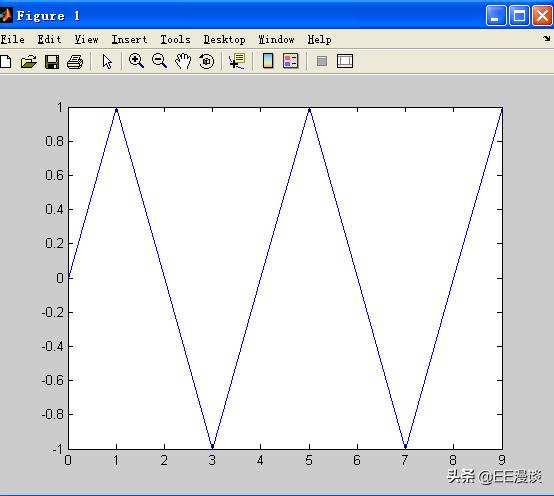现在函数式为x[n] = sin(n*pi/2),周期是4为什么看着不像以前的正弦函数了？因为这是对原函数抽样后得到的，而且它的横坐标已经变成了n而不是t。为了让抽样后的x[n]看起来更像x[t]那么方法只有一个，就是增加抽样率fs。当抽样率为80时x[n] = sin(n*pi/4),f0=10;fs=80;N=10;n=0:N-1;y=sin(2*pi*n*f0/fs);plot(n,y);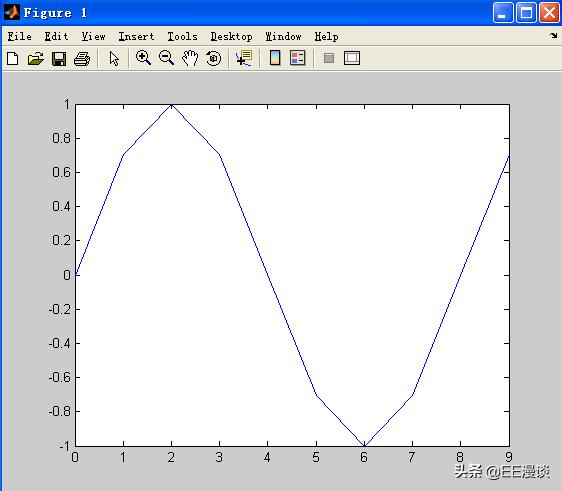看着更像x(t)了！这是因为抽样点更密了。所以产生x(t)=a*sin(2*pi*f0*t+ψ)的正弦波步骤：对x(t)进行以fs为抽样频率的抽样后得到x(n)=a*sin(2*pi*f0*n/fs+ψ)=a*sin(w*n+ψ)画的时候指明a，f0，fs，ψ就可以对任意sin函数进行画图那么，如何知道现在画出来的x(n)就是以前的x(t)呢？以前是T = 0.1S,现在变成了T = 8，在这种情况下如何换算呢？就有FFT了！下一篇文章我们再继续。
展开全文• 用于车辆平顺性仿真的路面随机激励时域模型，单轮车辆模型
• 有规则噪声的灰度图像快速傅里叶变换，观察去噪效果 定义 If it varies slowly, it is a low frequency signal. You can extend the same idea to images. Where does the amplitude varies drastically in images...
• 上一篇博客我们了解到，可以将一个...下面就讲讲如何实现傅里叶反变换傅里叶变换的函数是ifft()；但是ifft()有很多引用的参数语法讲究。 y = ifft(X) y = ifft(X,n) y = ifft(X,[],dim) y = ifft(X,n,dim)语音识别 函数 matlab
• 继 1900 年普朗克提出量子概念以后，出现了爱因斯坦的光电效应解释、玻尔 － 索末菲原子定态轨道理论(圆满解释了氢光谱线，但此理论与加速电子的辐射理论相抵触)、泡利的不相容原理(每一量子态不可能存在多于一个的...
• 而被其他频率混淆（干扰）的函数是不能完全通过反傅里叶变换还原回原函数的。 广义上的混淆 如果我们认为我们完全遵守奎更斯特（香农）定理，就万事大吉了。雷神高速我们：Too Young Too Simple. 因为在绝大多数的...
• 傅里叶反变换 函数 ： f = ifft2(F) 其中，F是傅里叶变换，f 是结果图。 因为 fft2 把输入图像毫无缩放的变为 double 类，因此解释反变换的结果时，必须小心。 例如，如果 f 是 uin8 类，则其值是范围 [0 255] 内...
• 这是用matlab处理的，半径为30的理想低通滤波器处理。... 这是自己写的程序，也是半径为30的理想低通滤波器处理的，但是...另外如果仅仅是把图像经过傅里叶变换后，不经过滤波而直接进行反傅里叶变换，能回复原来的图像。matlab 图像处理
• 1．熟悉傅立叶变换的各种性质 2．熟悉基本信号的频域转换 3．熟悉应用FFT对典型信号进行频谱分析的方法 4．熟悉在已经幅频函数|H(jw)|和相频函数arg(H(jw)的情况下，利用ifourier函数求傅里叶反变换得到的函数
• ==============功能描述：给定信号，对其进行傅里叶变换然后再进行反变换，绘制原始信号、傅里叶变换、傅里叶反变换的结果图像。给定信号，滤除其中某个频率范围的信号，绘制图像。参考代码：运行结果：温馨提示关注...
• 功能描述： ...给定信号，对其进行傅里叶变换然后再进行反变换，绘制原始信号、傅里叶变换、傅里叶反变换的结果图像。给定信号，滤除其中某个频率范围的信号，绘制图像。 参考代码： 运行结果： ...python
• 基于matlab的傅里叶变换与反变换的标准形式。
• 本文采用MATLAB软件编写程序,利用一维或二维的傅里叶变换函数,实现了对二维图像信息的傅里叶变换,通过程序对其频谱结果进行处理,利用傅里叶反变换函数,实现了对二维图像信息的压缩,进而展示了傅里叶变换与反变换在...
• 假设我有一组数据a=[1,2,3,4],我将其进行傅里叶变换，得到一组复数数据，我在将这组复数数据进行傅里叶反变换，是不是应该得到一组和a完全一样的数据。请高手帮忙给解答一下。 当然是的。。变换是为了...webbrowser schema include timer javascript
• ==============功能描述：给定信号，对其进行傅里叶变换然后再进行反变换，绘制原始信号、傅里叶变换、傅里叶反变换的结果图像。给定信号，滤除其中某个频率范围的信号，绘制图像。参考代码：运行结果：温馨提示关注......